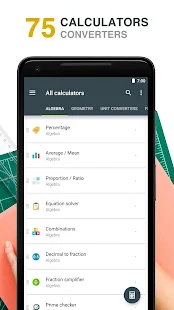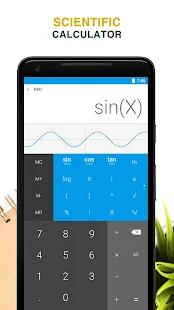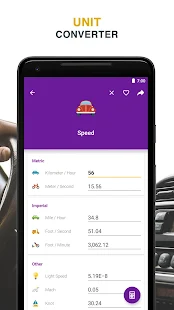# All-in-One Calculator v1.7.7 [Pro Mod]

Overview: All-In-One Calculator is a lightweight, clean and easy to use calculator and converter pack.
Containing over 50 calculators and unit converters packed in with a scientific calculator, it's the only math app you will ever need from now on on your device.

It's a great calculator for school and homework, but not only. If you're a scholar, student, teacher. builder, handyman, contractor, etc.. and you need an all in one tool for your device, give this one a try. You will never be disappointed.

Features
• Over 50 Calculators and Unit converters
• Scientific calculator with history
• Currency converter with 160 currencies, available offline
• Supports math formulas as input
• Instant results
• Integrated search
• Favorite list for quick access
• Tablet support

Languages: English (currently looking for translators)

Complete list of all the calculators and unit converters
Algebra
• Percentage calculator
• Proportion calculator
• Average calculator - arithmetic, geometric and harmonic means
• Equation solver - linear, quadratic and equation system.
• Combinations and permutations
• Decimal to fraction
• Fraction simplifier
• Greatest common factor & Lowest common multiple calculator
• Random number generator

Geometry
• Area / perimeter calculator for square, rectangle, parallelogram, trapezoid, rhombus, triangle, pentagon, hexagon, circle, circle arc, ellipse
• Volume calculator for cube, rect. prism, square pyramid, sq. pyramid frustum, trapezoidal footing, cylinder, cone, conical frustum, sphere, spherical cap, spherical frustum, ellipsoid.
• Right triangle calculator
• Heron's formula (solve a triangle knowing the side lengths)
• Circle solver

Unit converters
• Acceleration converter
• Angle converter
• Length converter
• Energy converter
• Force converter
• Torque converter
• Area converter
• Volume converter
• Volumetric flow converter
• Weight converter
• Temperature converter
• Pressure converter
• Power converter
• Speed converter
• Mileage converter
• Time converter
• Digital storage converter
• Data transfer speed converter
• Numeric base converter
• Roman numerals converter
• Shoe size converter

Finance
• Currency converter with 161 currencies available offline
• VAT calculator
• Tip calculator
• Loan calculator
• Electricity cost calculator
• Cost of smoking calculator

Health
• Body mass index - BMI
• Daily calories burn
• Body fat percentage

Engineering
• Ohm's law calculator - voltage, current, resistance and power
• Speed/Distance/Time
• Cylinder force calculator
• Resistance calculator
• Density calculator

Miscellaneous
• Elapsed time calculator

What's New:
Translation improvements and bug fixes.

Mod Info:
Pro features unlocked;
Disabled / Removed unwanted Permissions + Receivers and Services;
All ads and services calls from Activity removed;
Optimized graphics / Zipalign;
Analytics disabled.

••••